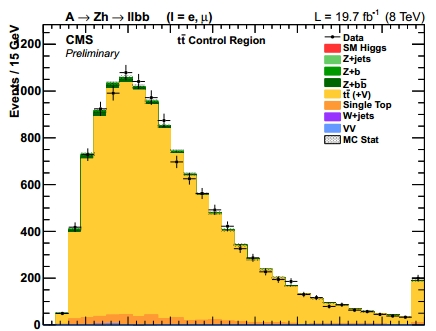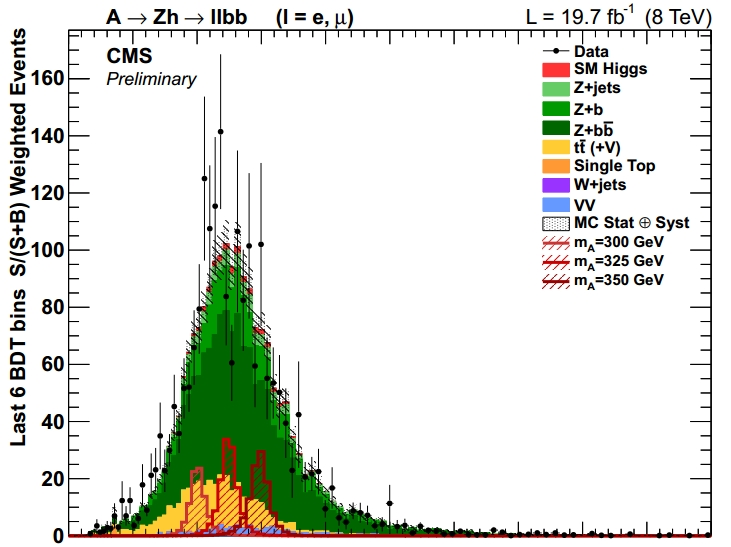## Searching for the A boson with CMS November 22, 2014

Posted by dorigo in physics.

I am quite happy to report today that the CMS experiment at the CERN Large Hadron Collider has just published a new search which fills a gap in studies of extended Higgs boson sectors. It is a search for the decay of the A boson into Zh pairs, where the Z in turn decays to an electron-positron or a muon-antimuon pair, and the h is assumed to be the 125 GeV Higgs and is sought for in its decay to b-quark pairs.

If you are short of time, this is the bottomline: no A boson is found in Run 1 CMS data, and limits are set in the parameter space of the relevant theories. But if you have a bit more time to spend here, let’s start with the beginning – What’s the A boson, you might wonder for a start.

The A particle is one of the five physical states resulting from the breaking of the electroweak symmetry by the Higgs mechanism, when instead of the minimal insertion in the standard model Lagrangian of a single complex doublet of scalar fields, one inserts two such doublets. The Higgs mechanism works quite similarly: instead of having plugged in four degrees of freedom with the complex doublet, three of which get absorbed by the positive and negative charged W and the Z boson, which all get mass terms in the Lagrangian, and one remains as The higgs boson, we have inserted eight degrees of freedom,  so we expect five higgs-like new states to appear.

Of the five higgses of two-doublet models, there are one positively and one negatively-charged one, two more scalars – the h and the H – and the pseudoscalar A. In the analysis it is assumed that h is the particle we found at 125 GeV two years ago. This typically makes the A heavy, although the space of parameters is quite complex and the phenomenology quite varied. As past searches of the A at lower energy have failed, the analysis concentrates on a mass range where the Zh final state is a possibility for the A disintegration: so the A is supposed to be heavier than 216 GeV -the total of Z and h masses.

CMS has collected 20 inverse femtobarns of proton-proton collisions at 8 TeV, and in that data sample there are tens of millions of Z boson decays to ee or μμ pairs. It is exactly there that the search starts. Then, two b-quark-tagged jets are sought in addition; the mass of the two b-jets is required to be close to 125 GeV; and a multi-variate algorithm is used to distinguish the selected data from backgrounds.

Data selection starts from millions of collision events and ends up with a sample of few thousands of them, where a possible A signal would be more easily seen. The initial selection includes two leptons from Z decay, then two jets events are kept, then only ones where the jets are b-tagged, and finally a cut is placed on the output of a  multi-variate algorithm (a BDT, boosted-decision trees) which distinguishes data from backgrounds using the distinctive features of the A decay kinematics.

As the two leptons and the two b-jets are supposed to come from the decay of a single particle, the four-body mass is the most distinguishing variable to look at. However, if you just combine the mass of the two leptons and two jets your mass resolution will be good but not great: jet energies, in particular, suffer from a 10% relative resolution which smears a bit the resulting peak from a resonance decay. What is done in the analysis is to fit the four 4-momenta to the hypothesis that the leptons come from the Z and the b’s come from the decay of the 125 GeV higgs. This “pulls” measured energies and momenta in the right direction and the final result is that the resolution on the four-body mass shrinks quite spectacularly, as you may check in the graph below.You can see several mass peaks, relative to different mass hypotheses for the A boson, before (dashed curves) and after (full curves) the application of the kinematical fit. It is clear that this strongly improves the chance to observe a signal in the data, especially if there is a large background -mostly due to Z+bb production and top production.

One of the nice things about the analysis is that in the final data sample all contributing backgrounds are tightly constrained in normalization from a global fit to a number of control regions simultaneously. There are control regions that specifically select top-rich events, Z+bb-rich events, Z+b-rich events, e.g.; so the fit is capable of correcting the simulation prediction for the yield of each of them. Below, for instance, is the missing ET distribution of the control region rich in top-pair decays: you can see that the yellow top contribution dominates the data, so a match of data and simulation in this control region strongly fixes the top contribution.In the end, the search for the signal is performed in the two-dimensional plane of 4-body mass and BDT output. This makes less easy to display the fit result, but one can produce projections, e.g. in the mass distribution. As the search is performed in a wide mass range, and the kinematics of the decay is strongly dependent on the A mass, three different BDTs are trained to select the signal. For the central mass region this is the mass projection:If you are wondering about the few high bins near 320 GeV, well, that’s a 2-sigmaish fluctuation, an effect which is entirely expected given the number of mass points that are independently investigated by the search. The result of the search is that there is no A boson in the data. Upper limits are derived in the context of two-doublet higgs models, and a series of such limits are obtained in the parameter space of the models. I refrain from showing those, as you can only appreciate them if you are an expert, and in that case I strongly suggest that you download the public CMS document describing the analysis, by paying a visit to the public web page of the analysis.